# Ellipse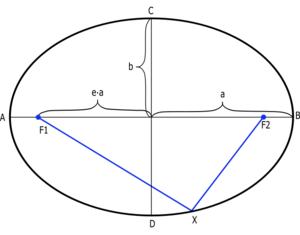In the figure, a is the semi-major axis, b is the semi-minor axis, F1 and F2 are the two focal points. The distance F1-X-F2 is constant.

An ellipse is a geometric figure that looks like a squashed circle. The more squashed it is, the greater its eccentricity. It is defined as the set of all points in a plane where the sum of the distance from two points, the foci, is the same. A special case of the ellipse is the circle, where the two points are coincident (in the same place). A circle has an eccentricity of 0. The terms eccentric and circular are antonyms.

Ellipses became important in astronomy in the early 1600s, when Kepler proved that planets revolving the sun always follow elliptical orbits, with the sun at one of the foci. This helped overturn the Ptolemaic theory, and led to Issac Newton's law of gravitation.

To construct an ellipse, place tacks at two points on a piece of paper with a string between them. Trace out the curve of the ellipse with a pencil which is always pushing against the string. The longer the string, the less eccentric the ellipse will be (and more circular).

## Mathematics

An ellipse is a conic section, the intersection of a plane and a cone, where the angle of the plane to the cone's axis is greater than the angle of the cone with its axis. The semi-major axis of an ellipse is half the longest diameter of the ellipse, while the semi-minor axis is half the shortest diameter of the ellipse.

The general algebraic formula for an ellipse in Cartesian coordinates is given by: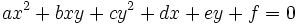For the case of an ellipse centred at the origin, the the semi-major axis along the x-axis, it can be written as,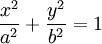where a is the semi-major axis and b is the semi-minor axis.

A pair of line segments drawn from one focal point of an ellipse to the curve and from there to its other focal point form an angle whose bifurcating line is always perpendicular to the tangent of the curve. This feature of an ellipse has been exploited in rooms having elliptical walls or (more dramatically) elliptical ceilings. A whisper at one focal point is easily heard at the other focal point, because the sound waves bounce off the walls and combine again at the other focal point.

### Area and Circumference

Unlike a circle, there is no closed form (simple equation) for the circumference of an ellipse. The area of an ellipse is given by: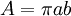where a is the semi-major axis and b is the semi-minor axis.

### Eccentricity

The eccentricity, e, of an ellipse can be computed from its semi-major and semi-minor axes as,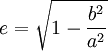Ellipses have eccentricites between 0≤e<1. A circle is a special case of an ellipse which has an eccentricity of zero.